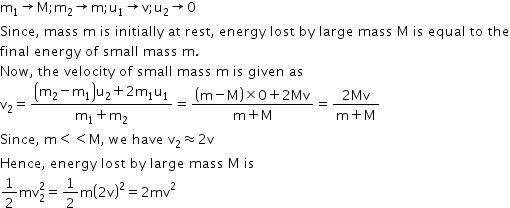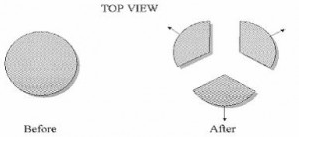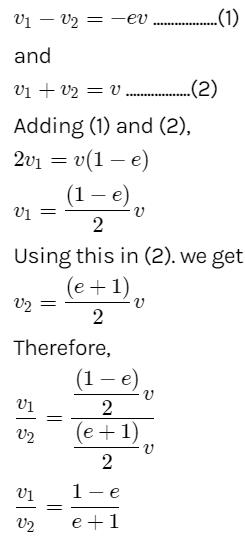Courses

# Test: Collisions

## 10 Questions MCQ Test Physics Class 11 | Test: Collisions

Description
This mock test of Test: Collisions for JEE helps you for every JEE entrance exam. This contains 10 Multiple Choice Questions for JEE Test: Collisions (mcq) to study with solutions a complete question bank. The solved questions answers in this Test: Collisions quiz give you a good mix of easy questions and tough questions. JEE students definitely take this Test: Collisions exercise for a better result in the exam. You can find other Test: Collisions extra questions, long questions & short questions for JEE on EduRev as well by searching above.
QUESTION: 1

### A bomb of mass 4 kg explodes in air into two pieces of masses 3 kg and 1 kg. The smaller mass goes at a speed of 90 m/s. The total energy imparted to two fragments is.

Solution:

By conservation of momentum we get the speed of the bigger part let say, v = 1 x90 / 3
Hence we get v = 30
Thus the total KE of the system after collision is ½ (3 X 900 + 1 X 8100)
Thus KE = ½ (10800) = 5400
Now  if we apply WET to the system, as no external force has acted upon it, we get
W = ΔKE
= 5400 - 0
= 5.4 kJ

QUESTION: 2

### In an elastic collision in one dimension if a body A collides against the body B of equal mass at rest, then the body A will

Solution:

Since collision is elastic and mass is same then after velocity exchange A body will stop and B will start moving with A's velocity in the same direction.

QUESTION: 3

### Which physical quantity is conserved during both elastic and inelastic collision?

Solution:

Momentum is conserved in all types of collision whether it is elastic or inelastic where as kinetic energy is lost in sound energy in the absence of external force in inelastic collision.

QUESTION: 4

A moving white hockey puck collides elastically with a stationary red hockey puck on a frictionless horizontal surface. No net external force acts on the two-puck system. Select all of the following statements that must be true for this elastic collision.

1. The kinetic energy of the white puck is conserved (same before and after the collision)
2. The linear momentum of the white puck is conserved.
3. The linear momentum of the two puck system is conserved.

Solution:

The angular momentum of the two pluck will remain conservated since no extra force is involved and elastically white hockey pluck collide with stationary red hockey pluck as its a frictionless horizontal surface pluck will keep moving forward with same speed

QUESTION: 5

A large mass M moving with velocity v makes an elastic head-on collision with a small mass m at rest. What will be the expression for energy lost by mass M?

Solution:QUESTION: 6

A bomb at rest on a horizontal frictionless surface explodes and breaks into three pieces that fly apart horizontally as shown below. Select all of the following statements that must be true after the bomb has exploded.

1. The total kinetic energy of the bomb fragments is the same as that of the bomb before explosion.
2. The total momentum of the bomb fragments is the same as that of the bomb before explosion.
3. The total momentum of all the bomb fragments together is zero.Solution:

So as no external force acts upon the system, we can say that momentum of the system remains conserved but as an internal force acts that explodes the bomb, the KE of the system is never conserved. But every fragment has some non zero momentum while the bomb initially has zero momentum.

QUESTION: 7

If a ball of mass 1 kg makes a head-on collision with a ball of an unknown mass initially at rest such that the ball rebounds to one third of its original speed, what is the mass of the other ball?

Solution:

As the balls collide head-on and no other external force is acting, momentum of the system realins conserve and so will be the energy of the system (i.e. KE)
Thus we get for the mass of other ball be m and initial velocity of mass 1 be v
1 x v = 1 x -v/3 + m x V         -1
For some V that is velocity of m after collision.
Also  ½ 1 x v2 =  ½ 1 x v2 /9 +  ½ m x V2                -2
Now from eq 1 we get mV = 4v/3
And from eq 2 we get  mV2  = 8v2 /9
Thus we get V =  (8v2 /9) / (4v/3)
=  2v/3
Thus we get m x 2v/3 = 4v/3
So m = 2kg

QUESTION: 8

When a ball is allowed to fall from a height of 20 m, 40% of its energy is lost due to impact. After one impact the ball will go up to a height of

Solution:

Before the impact the KE was ½ x m x (2g x 20) = 20mg
And let say v be the velocity after impact and for height h, v2= 2gh
Thus KE = ½ mv2 = ½m2gh = ⅗ x 20mg
Thus we get mgh = 12mg
thus h = 12 m

QUESTION: 9

A body of mass m moving with a constant velocity v hits another body of the same mass at rest and sticks to it. The velocity of the compound body after the collision is

Solution:

Correct Answer :- a

Explanation :Here, a body of mass m moving with a constant velocity v hits another body of the mass m moving with same velocity v but in the opposite direction, and sticks to it. We can write
m1​u1​−m2​u2​=(m1​+m2​)v
As m1​=m2​=m and u1​=u2​=v
2mv=0
v=0

QUESTION: 10

There is a sphere of mass m moving with constant velocity v. It hits another sphere of same mass which is at rest. If e is the coefficient of restitution, then the ratio of the velocities of the two spheres after collision will be

Solution:

Here, a sphere of mass m moving with a constant velocity v hits another stationary sphere of the same mass. As per the given problem we can write• Test